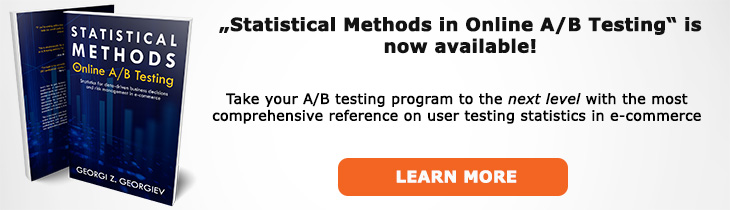# What does "Non-Binomial Metric" mean?

Definition of Non-Binomial Metric in the context of A/B testing (online controlled experiments).

## What is a Non-Binomial Metric?

Aliases: continuous metric

A non-binomial metric, a.k.a. continuous metric is any parameter which can take values on a continuum. Such a metric is non-discrete and can often span the entire real line while sometimes being limited from above or below (e.g. from 0 to +∞). Examples of such metrics are revenue, transactions, revenue per user, session duration, sessions per user, transactions per user as well as their averages (arithmetic means).

Unlike binomial metrics one needs to estimate the variance and standard deviation of continuous ones when performing sample size calculations / power analysis. Usually this is done by using historical data over a relevant period. It is often restricted to the past few months since often the more recent the data is the better its predictive value is for the actual standard deviation.

Similarly, when calculating a p-value or a confidence interval for a non-binomial metric one needs to estimate the population standard deviation from the sample. In an A/B test this usually involves computing the pooled standard deviation as a best estimator of population standard deviation under the null hypothesis. Unlike binomial metrics, for non-binomial metrics one needs the whole dataset in order to calculate the variance and standard deviation. For example, if a site had 10,000 users and 100 transactions during a certain period, if one wants to calculate statistics for average revenue per user they would require the whole 10,000 rows of data, most of which with revenue value of zero, in order to do so.

If one cares about statistics other than the arithmetic mean (average) one needs to use a non-parametric test if the distribution of the statistic is not normal. However, if one is interested in averages such as the average revenue per user (ARPU), average order value (AOV), average session duration, average sessions per user and so on, then, given the sample sizes usually present in online controlled experiments one is safe to use the traditional approaches that assume a normal distribution since the statistic of interest is then the standard error of the mean which is normally distributed in accordance with the Central Limit Theorem (CLT).

## Related A/B Testing terms

Binomial Metric

Average Revenue Per User

Average Order Value

## Articles on Non-Binomial Metric

Like this glossary entry? For an in-depth and comprehensive reading on A/B testing stats, check out the book "Statistical Methods in Online A/B Testing" by the author of this glossary, Georgi Georgiev.## Glossary Index by Letter

Select a letter to see all A/B testing terms starting with that letter or visit the Glossary homepage to see all.